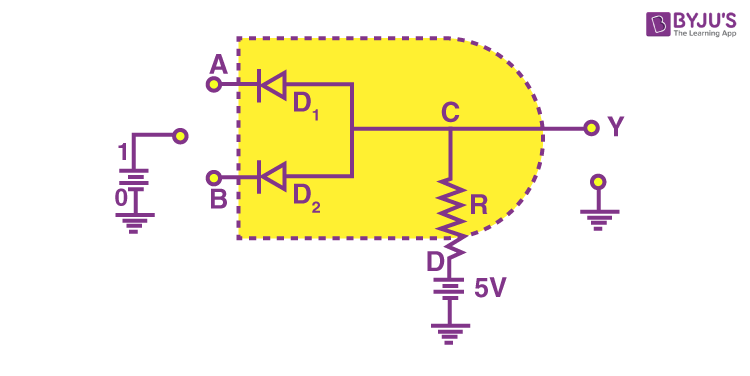# Draw the circuit diagram of AND gate using diodes.

AND gateAND gate is one type of logic gate in which the output of an AND gate attains the state 1 if and only if all the inputs are in state 1.

The Boolean expression of AND gate is Y = A.B

The truth table of a two-input AND basic gate is given as

 A B Y 0 0 0 0 1 0 1 0 0 1 1 1

Wired-AND connection(i) When both inputs are 1, the two diodes are reverse biased and there is no current flowing to the ground. So the output is 1 as there is no decrease in voltage around the R resistor.

(ii) When one of the logic inputs is ‘0’, the current flows through the corresponding diode and through the resistor. Thus, the ‘0’ logic will be the diode anode or the output.